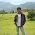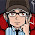## Wednesday, March 2

### Find the highest number in the array

'This code demonstrate how to find the highest number in a numaric array.

Dim num, i, Length1

num=array(34,12,98,43,89,49,56)
Length1 = UBound(num)    'Find the length of array

For i= 1 to Length1
If (num(i) < num(0)) Then  'to find lowest number, just change it to >
num(0)=num(i)
End If
Next

MsgBox num(0) 'Highest Number

1.thanks Abhikansh..
keep it up!! :)

2.outstanding work....keep it up....

3.Super!!

4.i tried to pass numbers(5,10,15) in array to find the biggest number and getting as 5. but it should be 15. pls help me in this.

num=array("5","10","15")
Length1 = UBound(num)
For i= 1 to Length1
If (num(i)>num(0)) Then
num(0)=num(i)
End If
Next

MsgBox num(0)

5.@raghav,

plz dont use double qoutes while paasing values in array..

6.superb! whatever search here i can get solution here thanks dude

7.8.plz help me... how to capture screen shot during runtime... what is syntax and explain plz

9.@satish k

plz search CaptureBitmap in qtp help

10.Good Job :)

11.Cool!

12.find the max value from array
Dim x,y,max
x=array(50,20,30,40,90)
z=UBound(x)
max=0
For i = 0 To z
If x(i)>max Then
max=x(i)
End If
Next
MsgBox max

13.Yo can try this one also:

Dim a, temp, i, j, firsthighest, secondhighest, thirdhighest
a = Array(35,44,99,66,98,76)
'Sorting the array in Ascending order
for i=0 to UBound(a)
for j=0 to UBound(a)
if (strcomp(a(i), a(j), 1)<0) then
temp = a(i)
a(i) = a(j)
a(j) = temp
end if
Next
Next

firsthighest = (UBound(a))
msgbox "First highest mark is: "&a(Firsthighest)

secondhighest = (UBound(a)-1)
msgbox "Second highest mark is: "&a(secondhighest)

14.I dint get these lines.....
num(i) is actually an number or index? why for loop started from 1 rather 0
For i= 1 to Length1
If (num(i)>num(0)) Then
num(0)=num(i)
End If
Next

15.The above code should be start little bit we need to correct
For i = 0 to Length

16.How can i sort larger number to lowest? but user input the numbers in arry